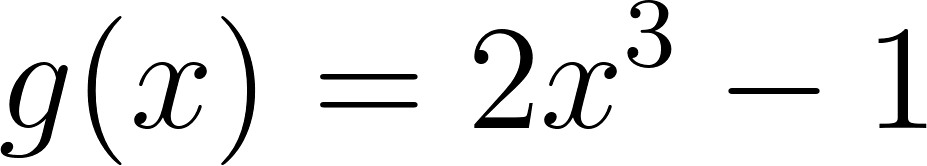×
Get Full Access to Calculus: Early Transcendentals - 1 Edition - Chapter 1.2 - Problem 34e
Get Full Access to Calculus: Early Transcendentals - 1 Edition - Chapter 1.2 - Problem 34e

×

# Solved: Shifting and scaling Use shifts and scalings toISBN: 9780321570567 2

## Solution for problem 34E Chapter 1.2

Calculus: Early Transcendentals | 1st Edition

• Textbook Solutions
• 2901 Step-by-step solutions solved by professors and subject experts
• Get 24/7 help from StudySoup virtual teaching assistantsCalculus: Early Transcendentals | 1st Edition

4 5 1 410 Reviews
22
5
Problem 34E

Shifting and Scaling:Use shifts and scalings to graph the given functions. Then check your work with a graphing utility. Be sure to identify an original function on which the shifts and scalings are performed.

Step-by-Step Solution:

Step 1 of 4

Given function,We need shifts and scalings to graph the given functions.

Step 2 of 4

Step 3 of 4

##### ISBN: 9780321570567

The full step-by-step solution to problem: 34E from chapter: 1.2 was answered by , our top Calculus solution expert on 03/03/17, 03:45PM. Calculus: Early Transcendentals was written by and is associated to the ISBN: 9780321570567. Since the solution to 34E from 1.2 chapter was answered, more than 367 students have viewed the full step-by-step answer. This full solution covers the following key subjects: scalings, shifts, performed, given, graph. This expansive textbook survival guide covers 85 chapters, and 5218 solutions. This textbook survival guide was created for the textbook: Calculus: Early Transcendentals, edition: 1. The answer to “?Shifting and Scaling:Use shifts and scalings to graph the given functions. Then check your work with a graphing utility. Be sure to identify an original function on which the shifts and scalings are performed .” is broken down into a number of easy to follow steps, and 35 words.

Unlock Textbook Solution